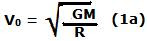## Sunday, November 9, 2008

### Irodov Problem 1.228

When an object is rotating at a distance r in a stable circular orbit around a planet of mass M, its velocity vr is given by,If the body is orbiting very close to the palnet's surface then its orbital velocity will be,The total energy (sum of potential energy and kinetic energy) of a body that cannot escape from the gravitational force of a planet is always negative since its negative potential energy exceeds the positive kinetic energy. For any body to be free from the influence of the gravitational field of a planet it needs to have a total positive energy, in other words its kinetic energy must exceed its negative potential energy. Escape velocity is the minimum velocity which is needed by a body to escape the gravitational force of a planet from its surface. The total energy of a body that has escape velocity is 0, since with this minimum kinetic energy it just manages to escape from the planets gravitational force. So if the planets radius is R, then,Now knowing that,We can compute the orbital and escape velocities of moon as 1.68 Km/s and 2.377 Km/s. For Earth they are, 7.91 Km/s and 11.186 Km/s.